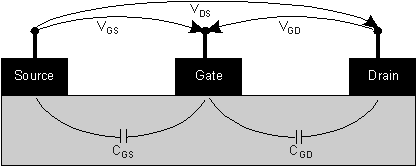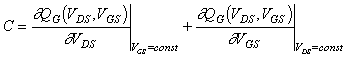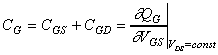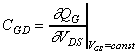Next: 4 Description of the Simulator Up: 3 Measurement and Parameter Extraction Previous: 3.2 Small Signal Equivalent Circuit Parameter Extraction

3.3 Determination of Capacitances by Quasi Static Approximation

The capacitances shown in  Figure 3.1 are considered to be frequency independent. Thus, they can be determined by a quasi static approximation. The voltages on the device are defined in  Figure 3.3.Figure 3.3 Definition of voltages and capacitances of a FET.

The charge on the gate is a function of two voltages, therefore the capacitance can be written in the general form. (13)

If the transfer characteristics of a FET is considered VDS is kept constant and the first part in (13) is zero. In this case VGS and therefore also VGD is changed. This way the total gate capacitance CG = (CGS+CGD) is determined by. (14)

Considering the output characteristics of a FET VDS is changed and VGS is kept constant, therefore the second part in (13) is zero and only CGD is given by. (15)

Thus, CGS is determined by CGS = CG - CGD.

Helmut Brech
1998-03-11